# Range Condition Stocking Rate Range Condition Determining the

• Slides: 16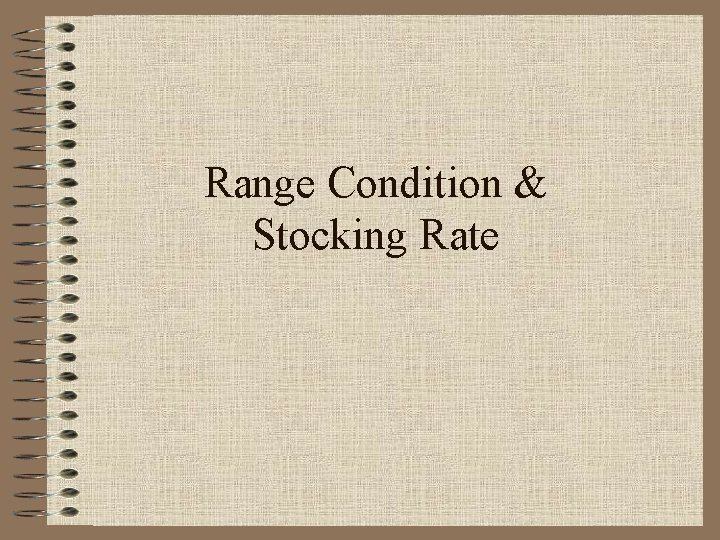Range Condition & Stocking RateRange Condition • Determining the health of a range • Need to know: – Region (Eastern Glaciated Plains) – Range Site (Thin Silty) – Plants Present and amounts of each in percent • Place plants in correct categories on worksheet • Rule out any invaders • Determine maximum allowable per plant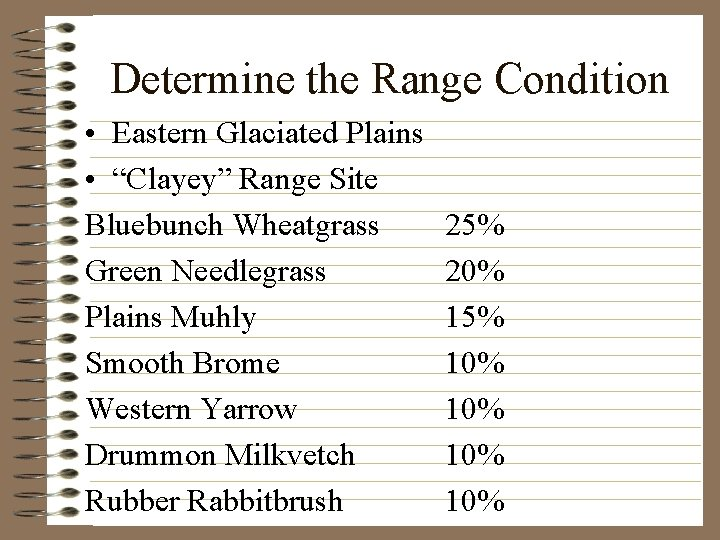Determine the Range Condition • Eastern Glaciated Plains • “Clayey” Range Site Bluebunch Wheatgrass Green Needlegrass Plains Muhly Smooth Brome Western Yarrow Drummon Milkvetch Rubber Rabbitbrush 25% 20% 15% 10% 10%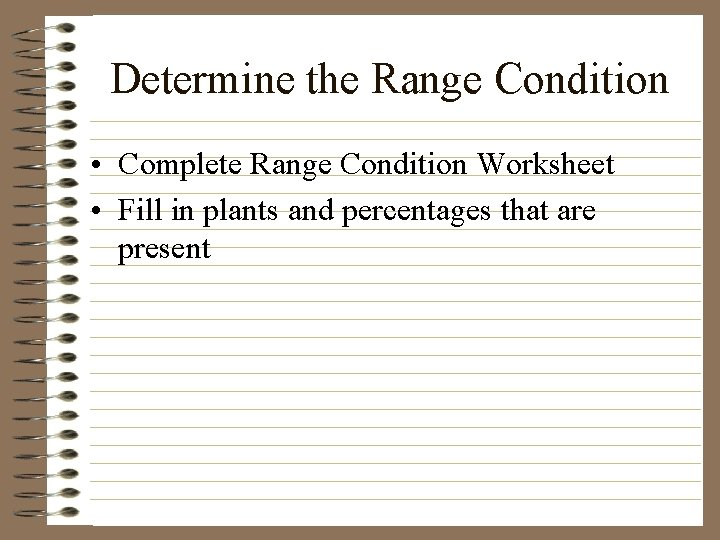Determine the Range Condition • Complete Range Condition Worksheet • Fill in plants and percentages that are present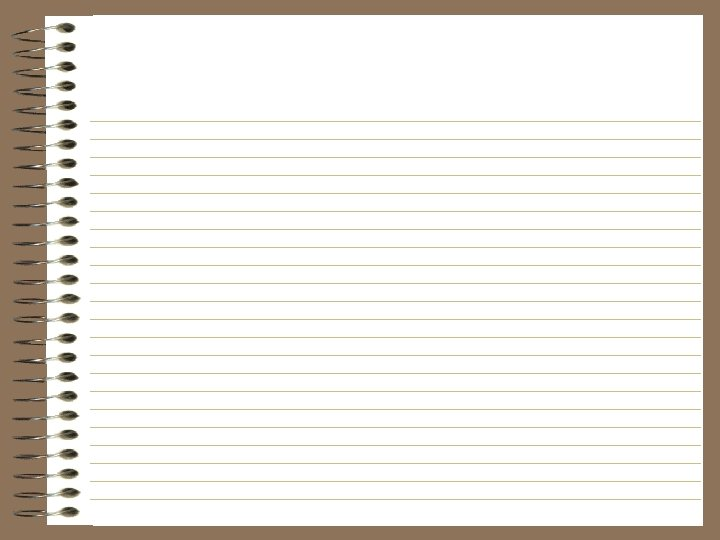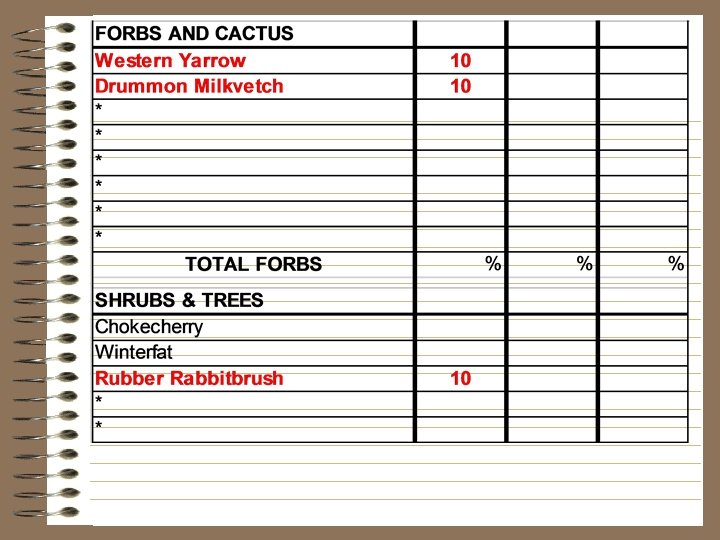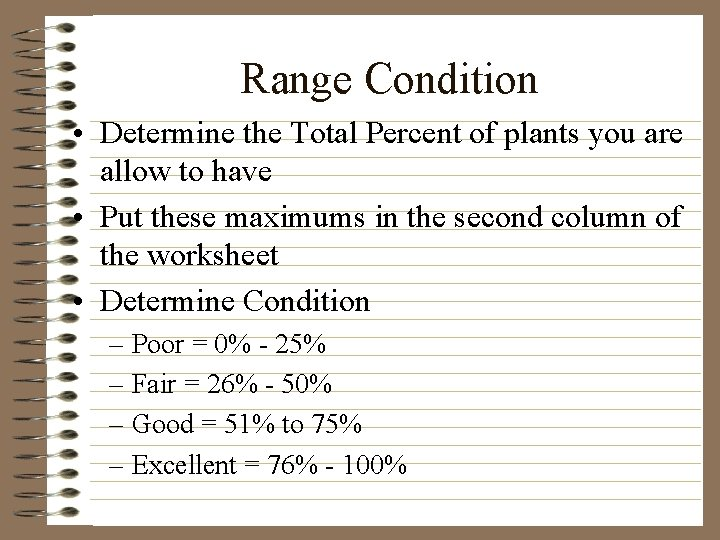Range Condition • Determine the Total Percent of plants you are allow to have • Put these maximums in the second column of the worksheet • Determine Condition – Poor = 0% - 25% – Fair = 26% - 50% – Good = 51% to 75% – Excellent = 76% - 100%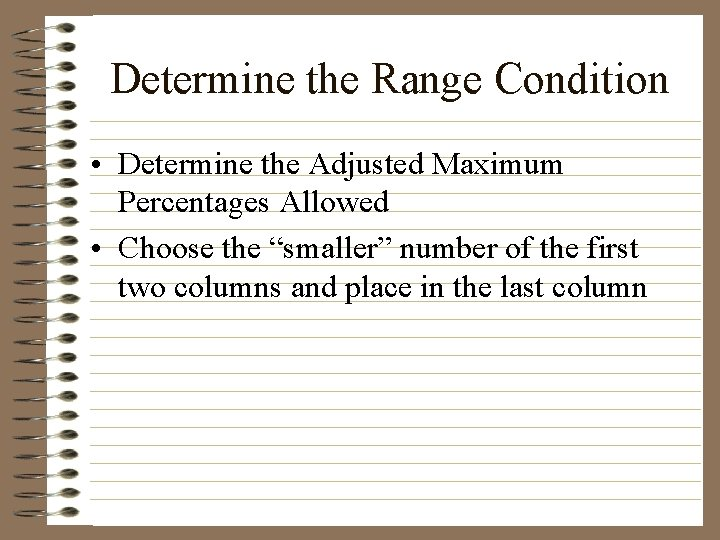Determine the Range Condition • Determine the Adjusted Maximum Percentages Allowed • Choose the “smaller” number of the first two columns and place in the last column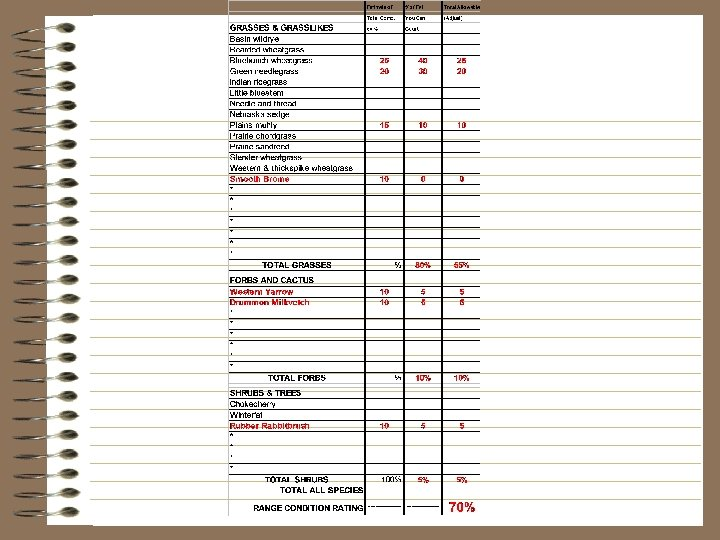Determine the Range Condition • Determine Range Condition Rating – 70% • Determine the Range Condition – Good • Complete Stocking Rate Problems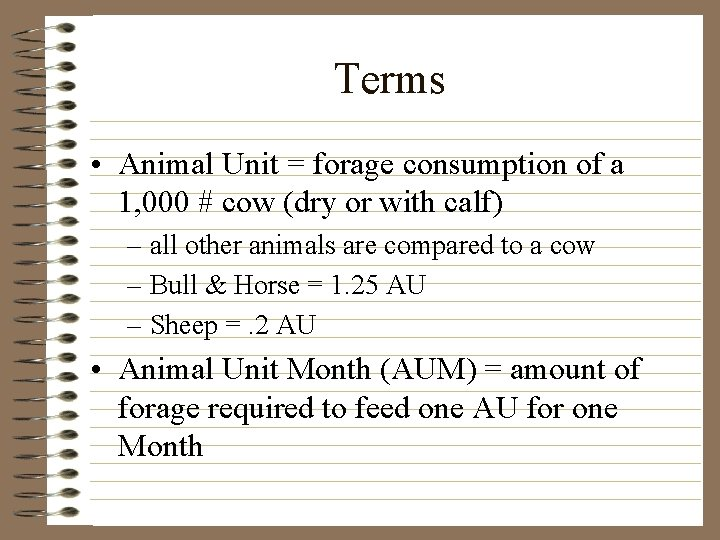Terms • Animal Unit = forage consumption of a 1, 000 # cow (dry or with calf) – all other animals are compared to a cow – Bull & Horse = 1. 25 AU – Sheep =. 2 AU • Animal Unit Month (AUM) = amount of forage required to feed one AU for one Month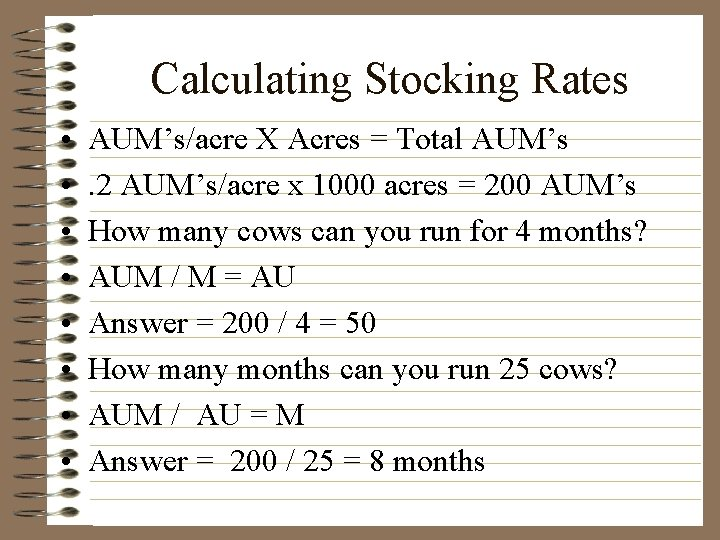Calculating Stocking Rates • • AUM’s/acre X Acres = Total AUM’s. 2 AUM’s/acre x 1000 acres = 200 AUM’s How many cows can you run for 4 months? AUM / M = AU Answer = 200 / 4 = 50 How many months can you run 25 cows? AUM / AU = M Answer = 200 / 25 = 8 monthsCalculating Stocking Rates • How many months for a herd of 50 sheep and 10 cows? • AUM / AU = M • 200 / ((50 x. 2) + (10 x 1)) = 10 • How many cows along with 4 horses for 2 months? • AUM / M = AU • 200 / 2 = 100 AU • 100 AU = (4 x 1. 25) + cows • 100 - 5 = 95 cows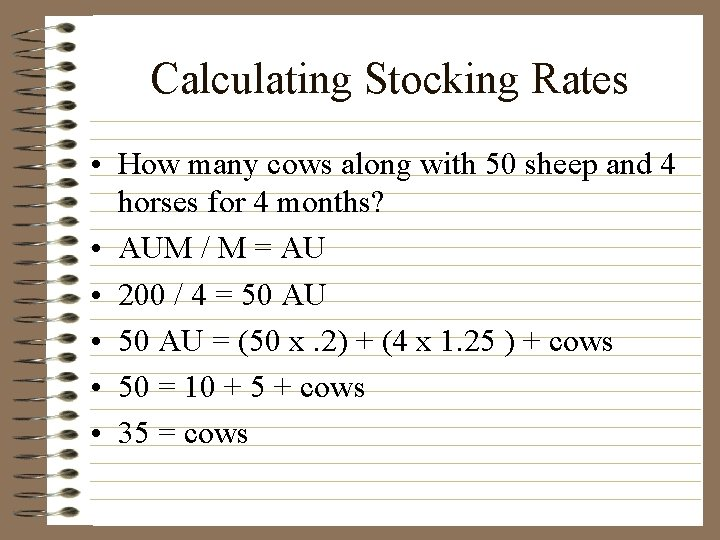Calculating Stocking Rates • How many cows along with 50 sheep and 4 horses for 4 months? • AUM / M = AU • 200 / 4 = 50 AU • 50 AU = (50 x. 2) + (4 x 1. 25 ) + cows • 50 = 10 + 5 + cows • 35 = cows